# Tokyo 2020 Betting I: Predictive Models for Pairwise Matches

The first in a series of posts building towards betting on the track cycling at the Tokyo Olympics. This post introduces the series, and the basic model behind my attempt to win big at the bookies!

This is the first post in a series: Click for links

Tokyo 2020 Betting II: Model Refinement and Feature Engineering

Tokyo 2020 Betting III: From Matches to Medals… and Bookies

The Olympics are officially under way, and I’m primed to become an armchair expert in sports that I haven’t expressed any interest in for four years. As a proactive way to engage with the games I thought I’d explore how to integrate predictive modelling with betting strategies.

Statistical models are common place amongst bookmakers, and I’m not naive enough to believe a model I threw together over a few days could beat a concerted effort by experienced sports analysts. However, I first explored this project for the 2019 Track Cycling World Championships, but UK bookmakers weren’t offering odds, let alone those derived from machine learning: so that gives me hope that a team of dedicated track cyling modelers simply doesn’t exist!

I’m tentatively optimistic odds will be available this time around, what with it being the olympics. It’d be particularly fitting given cycling is one of only four legal betting markets in Japan.

In this post I’ll provide a brief introduction to the event I’m hoping to bet on, and the statistical model which will form the base of my strategy.

Subsequent posts will refine the model through feature engineering, and derive the betting strategy itself. If the bookies play-ball and provide odds, I’ll place some bets and summarise how this experiment turned out!

I’m also hoping this series will serve as an example of my analytical workflow: to that end I’ll explain some of the errors and simplifications I made along the way, how I spotted and resolved them.

Not least I’ll say up front this work might not yield usable odds! Like many predictive models, this one is susceptible to Covid 19 impacts: most of the track cycling calendar for 2020 and 2021 was cancelled so we simply don’t have much recent data.

But I won’t let that spoil the fun, after all you can’t win if you don’t play the game…

### Assumptions

This post makes some assumptions about you: that you have some prior exposure to (or are keen to learn) regression, and evaluation metrics for classification models.

Specifically I’ll assume you’re familiar with logistic regression and evaluating predictive performance with accuracy and log-loss.

If you’re interested in reading the underlying code, this is in R and Stan.

# Track Sprinting and Data Overview

A rule of thumb in modelling the outcome of sports matches is that rather than predicting binary win/lose probabilities, its better to predict expected score (or time) differences and infer the winner from this.

As Andrew Gelman puts it (in the context of election modelling): there’s a lot of information in the score (or vote) differential that’s thrown away if you just look at win/loss.

The Individual Sprint discipline in track cycling is interesting from a modelling perspective, as it forces us to diverge from this wisdom.

The sprint is contested by two athletes with the winner being the first across the line. Unlike its track and field equivalent, the sprint isn’t contested from the start: the race starts slowly with a tactical game of cat-and-mouse, before one rider tries to catch the other unaware and starts their sprint. This dynamic renders race times meaningless for prediction.

Detailed match results for most large track cycling events are maintained by Tissot. These results are made available in a common PDF format, that I have parsed to extract structured data from.

The data I’m using spans ~4 years from November 2017 to July 2021, and for this post I’ll focus on the women’s competition. During that period I have data for a total of 610 matches between 123 athletes.

The sample below shows the fields that are immediately relevant to the initial model; I’ll explore the predictive strength of further information in the next post.

Field Example
event 2020 UCI TRACK WORLD CYCLING CHAMPIONSHIPS
date 2020-02-28
gender Women
round Finals
rider_1 HINZE EMMA
rider_2 VOINOVA ANASTASIIA
rider_id_1 44
rider_id_2 123
winner_id 44
loser_id 123

In all model runs I’ll use data up to the end of 2019 for training, and then evaluate against data from 2020 onward: a total of 1118 matches in the training data, and 176 held out for evaluation.

The Bradley-Terry model is a well studied approach to paired comparisons, and particularly to win/lose matches, which originates in work by Zermelo, who used it to compare chess players abilities.

The model was re-discovered and popularised by Bradley and Terry in the 1950s - their example application is somewhat more esoteric, as they used it to rank pork roasts!

I’ll introduce the model in the context of athletes competing in the Individual Sprint. Each of $$R$$ athletes involved in the league are assumed to have some strength $$\beta_r$$, $$r = 1,\ldots, R$$, that describes their ability.

The Bradley-Terry model assumes that a rider $$r$$ beats $$s$$ with probability

$\mathbf P \left[ r \text{ beats } s\right] = \frac{\beta_r}{\beta_r + \beta_s}.$

The aim is to estimate the strength parameters from historical matches, and then use these to predict future games.

One useful step is to change the scale of the strength parameters, considering $$\alpha = \log \beta$$, which changes the model to

\begin{align*} \mathbf P \left[ r \text{ beats } s\right] & = \frac{e^{\alpha_r}}{e^{\alpha_r} + e^{\alpha_s}} \\ & = \frac{e^{\alpha_r - \alpha_s}}{1 + e^{\alpha_r - \alpha_s}} \\ & = \text{logit}^{-1}\big(\alpha_r - \alpha_s\big) \end{align*}

This transformation has converted a complex fractional relationship between rider strengths into a linear relationship, inside a link function.

Specifically, this model is now equivalent to a classic logistic regression problem. To see this more clearly we can look at how we might implement this in code:

We create a data frame with a row per match, and $$R + 1$$ columns. For a match betwee n riders $$r$$ and $$s$$the row will have entries of 0 in each of the rider columns, except for an entry of +1 in the column for $$r$$, and -1 for $$s$$. The result column is set to 1 if the rider marked as +1 wins, and 0 if -1 wins.

Passing this data to your favouritetool for logistic regression (eg. glm in R) will fit the Bradley-Terry model as its defined above. Under the hood this will run an optimisation algorithm to find the vector of strengths $$\alpha^*$$ that maximises the likelihood.

There is a challenge however, as this solution won’t be unique: any translation by a constant, $$\alpha^* + C$$ will have the same likelihood. In the frequentist approach this is resolved by introducing a constraint that $$\sum_r \alpha_r = 0$$.

Rather than following the frequentist approach, I’ll fit a Bayesian Bradley-Terry model. My general rationale for working with Bayesian models is here, but in this instance there’s an added incentive as it opens an alternative method to resolving the identifiability issue above.

The idea is that rather than forcing the coefficients to sum to 0, we can use priors on the rider strengths that helps the likelihood to focus on one specific translation over all others. In our case we’ll assume athlete strengths are normally distributed, with mean $$0$$, which will lean the model towards configurations with $$\sum_r \alpha_r = 0$$, without the need to code up this constraint explicitly.

Rather than specifying the standard deviation for the prior I will treat this as an unknown hyperparameter, $$\sigma$$. This is known as partial pooling, or hierarchical effects, and has the effect of ensuring the athlete strengths are on a common scale.

The prior for $$\sigma$$ needs to be constrained to be positive (since standard deviation is positive), and for now I’ll pick a common default of a half-normal distribution, with variance of 1. Putting this together we have the following model specification:

BT1 \begin{align*} \bf{\text{Priors}}\\ \sigma & \sim \text{Half-Normal}(0,1) \\ \alpha_r & \sim \text{Normal}(0,\sigma) \\ \\ \bf{\text{Likelihood}}\\ \alpha_r - \alpha_s | W_{r,s} &\sim \text{logit}^{-1}(\alpha_r - \alpha_s) \end{align*}

Example Data
Show code
matches_sample <-  matches %>%
filter(event == '2020 UCI TRACK WORLD CYCLING CHAMPIONSHIPS', round == 'Finals', gender == 'Women') %>%
mutate(across(everything(), as.character)) %>%
select(event, date, gender, round, rider_1,rider_2,rider_id_1,rider_id_2, winner_id,loser_id) %>%
slice(1)

matches_sample <- tibble(Field = names(matches_sample), Example = unlist(matches_sample[1,]))
matches_sample %>%
kable("pipe") %>%
kable_styling(full_width=FALSE, bootstrap_options = c("striped", "hover", "condensed"),font_size = 10)

Field Example
event 2020 UCI TRACK WORLD CYCLING CHAMPIONSHIPS
date 2020-02-28
gender Women
round Finals
rider_1 HINZE EMMA
rider_2 VOINOVA ANASTASIIA
rider_id_1 44
rider_id_2 123
winner_id 44
loser_id 123

Stan code

writeLines(readLines(paste0(remote_project_path, 'stan/bt1.stan')))


functions {
real accuracy(vector delta, int start, int end){
vector[end - start + 1] delta_seg = segment(delta, start, end - start + 1);
real acc = 0;

for(n in 1:num_elements(delta_seg)) acc += (delta_seg[n] > 0);

return inv(num_elements(delta_seg)) * acc;
}

real log_loss(vector delta, int start, int end){
vector[end - start + 1] delta_seg = segment(delta, start, end - start + 1);
real ll = 0;

return inv(num_elements(delta_seg)) * bernoulli_logit_lpmf(1 | delta_seg);
}
}

data {
// Dimensions
int<lower=0> R; // Riders
int<lower=0> M; // Matches
int<lower=0,upper = M> T; // Training matches

// Match results
int<lower=1,upper=R> winner_id[M]; // ID's specifying riders in match
int<lower=1,upper=R> loser_id[M];
}

parameters {
// rider ratings
real<lower=0> sigma;
vector[R] alpha0;
}

transformed parameters {
// difference of winner and loser rating
vector[M] delta =alpha0[winner_id] - alpha0[loser_id];
}

model {
// (hierarchical) priors - strength
sigma ~ normal(0,1);
alpha0 ~ normal(0,sigma);

// likelihood
}

generated quantities {
// maximum theoretical strength difference between two riders
real<lower=0> delta_max = max(alpha0) - min(alpha0);

// Calculate log-loss, match log-loss and accuracy. A separate value is returned
// for training/evaluation data, and within each of these the metrics are further
// broken down by round (5 rounds in total) and the total (6th entry in vectors).
real training_accuracy;
real evaluation_accuracy;
real training_log_loss;
real evaluation_log_loss;

training_accuracy = accuracy(delta,1, T);
training_log_loss = log_loss(delta, 1,T);
evaluation_accuracy = accuracy(delta, T+1, M);
evaluation_log_loss = log_loss(delta, T+1, M);
}

Throughout this series I’ll fit the models using Stan. The code for the model is available in the tab above. In these posts I’ll focus less on the implementation, and more on the outputs of the models.

The plot below shows the posterior 90% credible intervals for each rider’s strength, for clarity I’ve filtered the data down to those riders who have competed since the start of 2020.

Show code
riders <- read_remote_target("riders_Women")

bt1_strength_summ <- bt1_summ %>%
filter(str_detect(variable, 'alpha')) %>%
mutate(rider_id = str_match(variable, '\$(.*)\$')[,2] %>% as.numeric()) %>%
left_join(riders) %>%
mutate(rider_name = fct_reorder(rider_name,median)) %>%
filter(max_date >= ymd(20200101))

p_strengths_bt1 <- ggplot(bt1_strength_summ) +
geom_segment(aes(x=q5,xend=q95,y=rider_name,yend=rider_name), color = infreq_palette["darkblue"]) +
labs(y="",x="Strength, α")

p_strengths_bt1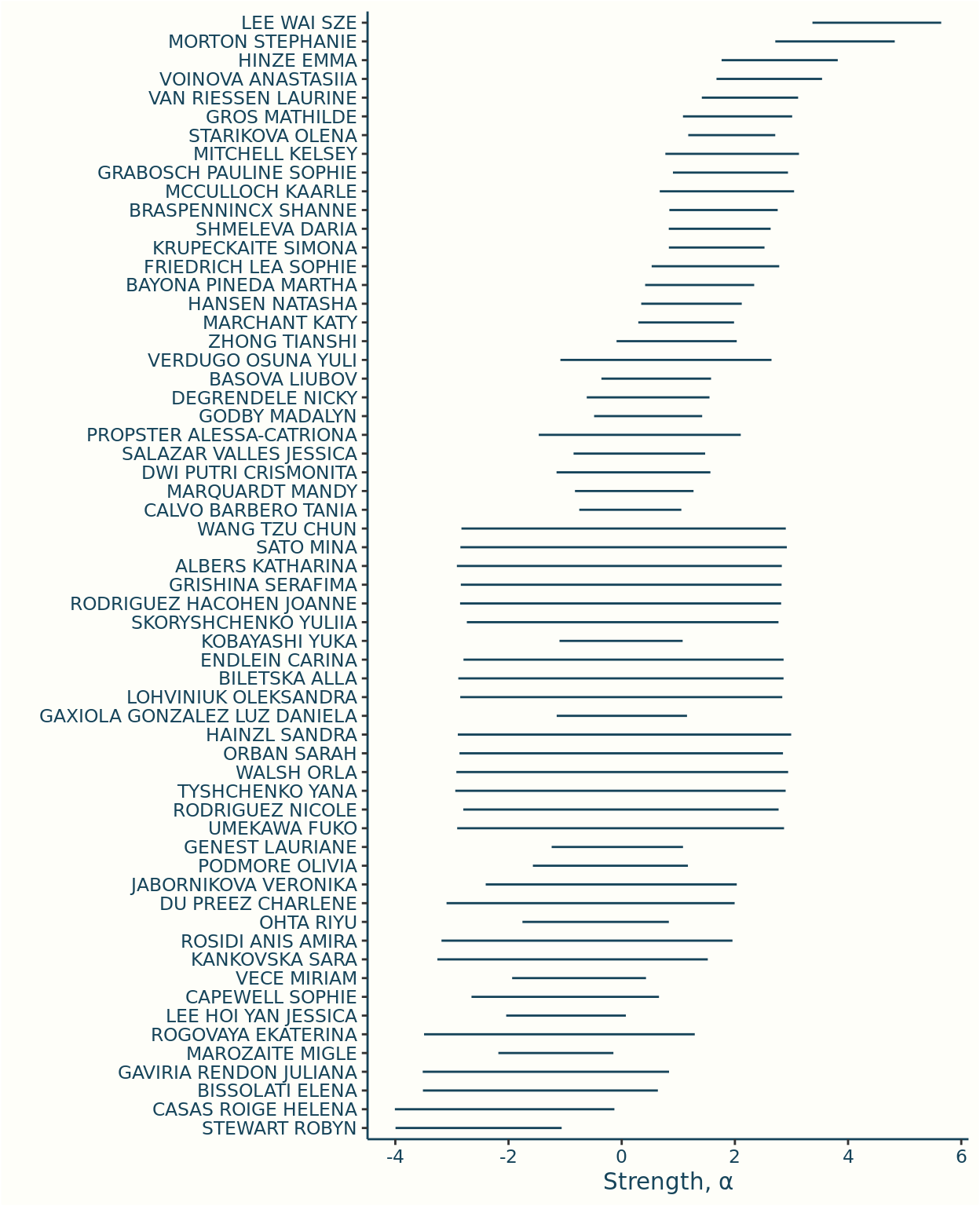From a first pass the strengths seem to fit what we might expect: for instance the top four riders have all appeared in the Finals of the World Championships in either 2019 or 2020.

We can also see riders, such as Nicole Rodriguez, who appear in the evaluation date but not the training data: they have wider uncertainty intervals as the model can only assume they are average in absence of any data.

The next question is whether the scale of the strengths looks right. A good test scenario for this is to compare the weakest and strongest riders: suppose those two riders were to compete, what odds would we put on the weaker rider winning?

The table below summarises the posterior odds for that extremal match

Show code
bt1_max_odds <- bt1_summ %>%
filter(str_detect(variable, 'delta_max')) %>%
select( q5, median, q95) %>%
mutate(
# calculation is based on the fact that the strength difference is the log odds
# on the exponential scale
across(everything(), ~format(round(10^(./log(10)),-2),big.mark = ","))
)

bt1_max_odds %>%
kable(col.names = c( "5%", "50%", "95%"), digits = 2) %>%
kable_styling(full_width=FALSE)

5% 50% 95%
1,500 12,000 211,900

The model is incredibly sceptical that the weakest rider could beat the strongest: I certainly can’t imagine a bookmaker offering odds on these scales!

Does the data genuinely support such high odds, or is this behaviour being encouraged by the somewhat arbitrary choice of prior distribution?

# Prior Predictive Checks

Prior predictive checks provide results from the model in absence of any data: i.e. using only our prior assumptions. They provide a good test for whether the model is sensibly defined, and in particular whether the prior distributions you’re using are suitable.

The plot below shows the prior distribution for the odds that the weakest rider beats the strongest.

Show code
sigma_draws_halfnormal <-
tibble(draw = 1:10000, hyperprior = 'HalfNormal(0,1)') %>% mutate(sigma = abs(rnorm(n())))

prior_draws_halfnormal <- crossing(rider = 1:nrow(riders), sigma_draws_halfnormal) %>%
mutate(alpha = rnorm(n(), sd = sigma))

max_diff_halfnormal <- prior_draws_halfnormal %>%
group_by(draw,hyperprior) %>%
summarise(
alpha_max = max(alpha),
alpha_min = min(alpha),
max_diff = alpha_max - alpha_min,
odds_weaker_wins_log10 = max_diff / log(10)
) %>%
ungroup()

ggplot(max_diff_halfnormal, aes(odds_weaker_wins_log10)) + geom_histogram(binwidth = 0.1, color = infreq_palette["beige"]) +
scale_x_continuous(breaks = seq(0,8,by = 2), labels = scales::math_format(10^.x)) +
labs(x = 'Odds Weakest Beats Strongest', y = '')  +
theme(axis.ticks.y = element_blank(), axis.text.y=element_blank(), axis.line.y = element_blank())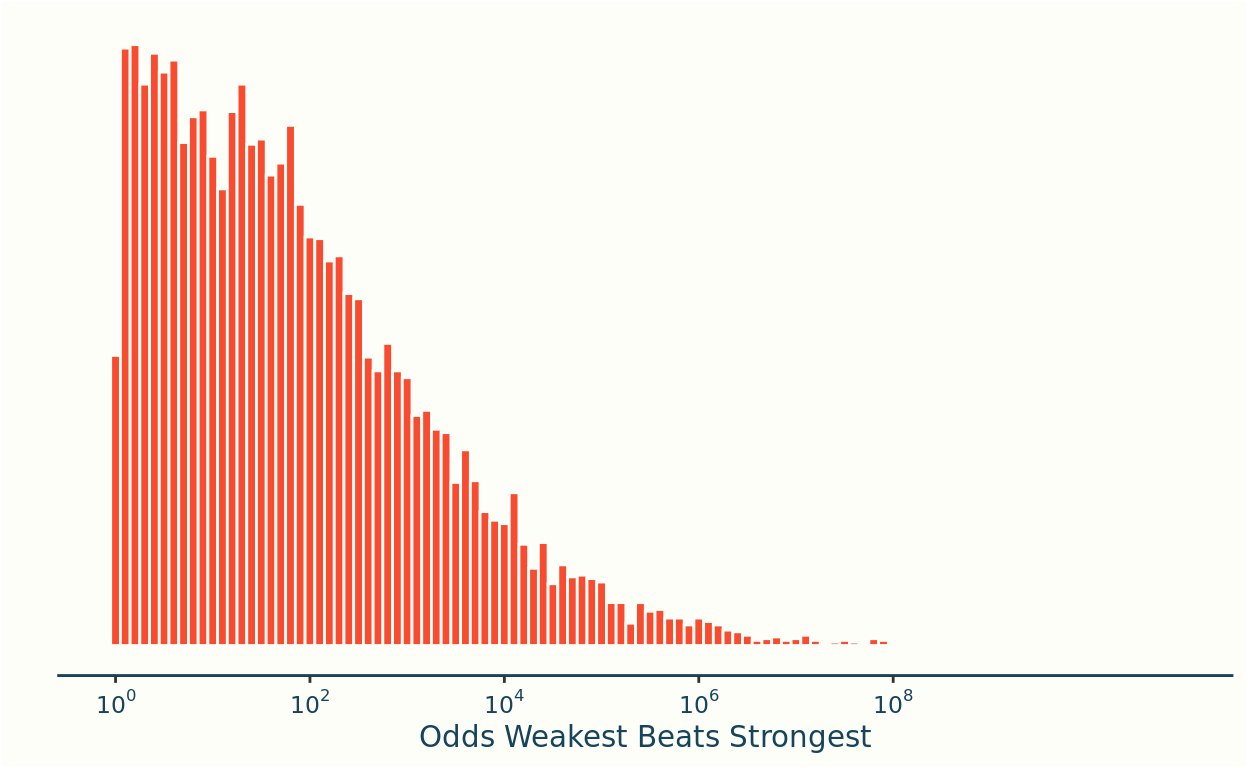The prior spans several orders of magnitude: putting mass on odds from 1-1, up to 1-1,000,000.

In hindsight this feels too vague, and the long tail might be promoting the model to tend towards extreme scenarios.

# An Informative Hyperprior

My personal instinct is that the odds should be more in the range of 1-100 up to 1-1,000, so as a first model development I’ll introduce a more informative prior to reflect my personal intuition about the scale of the odds.

Since the model is defined in terms of strengths I’ll need to convert that range of 1-100 to 1-1,000 odds to a range for the difference in strengths. In practice this conversion is almost immediate as the strength differences are none-other than the (base-$$e$$) logarithm of the odds. That’s just the magic of logistic regression!

So my preferred odds range of between 1-100 and 1-1,000 translates to a maximum difference in athlete’s strength of between 4.6 and 6.9.

I’ve decided to work with a Gamma distribution for the hyperprior, and settled on shape and rate parameters of 80, and 60 respectively: these were chosen by trial and error, to create a prior distribution that nicely spanned the strength range above.

σ - Hyperprior
Show code
sigma_draws_gamma <- tibble(draw = 1:10000, hyperprior = 'Gamma(80,60)') %>% mutate(sigma = rgamma(n(), 80, 60))

sigma_draws <- bind_rows(sigma_draws_gamma, sigma_draws_halfnormal)

ggplot(sigma_draws, aes(sigma,fill=hyperprior)) +
geom_histogram(aes(y=..density..),binwidth = 0.05, color = infreq_palette["beige"]) +
scale_x_continuous(limits = c(0,3)) +
facet_grid(rows = vars(hyperprior)) +
labs(x = 'σ', y = '') +
theme(
axis.ticks.y = element_blank(), axis.text.y=element_blank(), axis.line.y = element_blank(),
strip.background = element_blank(), strip.text = element_blank(), legend.title = element_blank()
)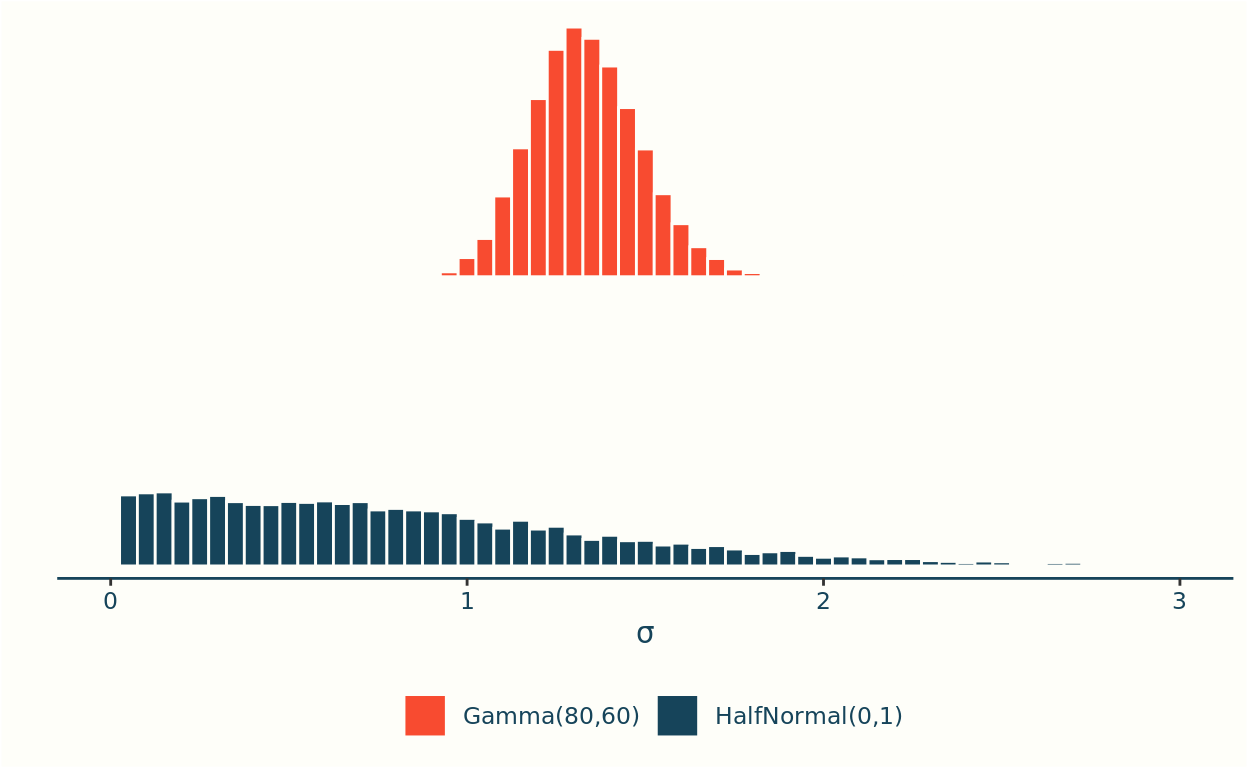Prior on Maximum Strength Difference

Show code
prior_draws_gamma <- crossing(rider = 1:nrow(riders), sigma_draws_gamma) %>%
mutate(alpha = rnorm(n(), sd = sigma))

max_diff_gamma <- prior_draws_gamma %>%
group_by(draw,hyperprior) %>%
summarise(
alpha_max = max(alpha),
alpha_min = min(alpha),
max_diff = alpha_max - alpha_min,
odds_weaker_wins_log10 = max_diff / log(10)
)

max_diff <- bind_rows(max_diff_gamma, max_diff_halfnormal)

ggplot(max_diff, aes(max_diff,fill=hyperprior)) +
geom_histogram(aes(y=..density..),binwidth = 0.25, color = infreq_palette["beige"]) +
scale_x_continuous(limits = c(0,15)) +
facet_grid(rows = vars(hyperprior)) +
# scale_x_continuous(breaks = seq(0,8,by = 2), labels = paste0("10^",seq(0,8,by = 2) )) +
labs(x = 'Max. Strength Difference', y = '') +
theme(
axis.ticks.y = element_blank(), axis.text.y=element_blank(), axis.line.y = element_blank(),
strip.background = element_blank(), strip.text = element_blank(), legend.title = element_blank()
)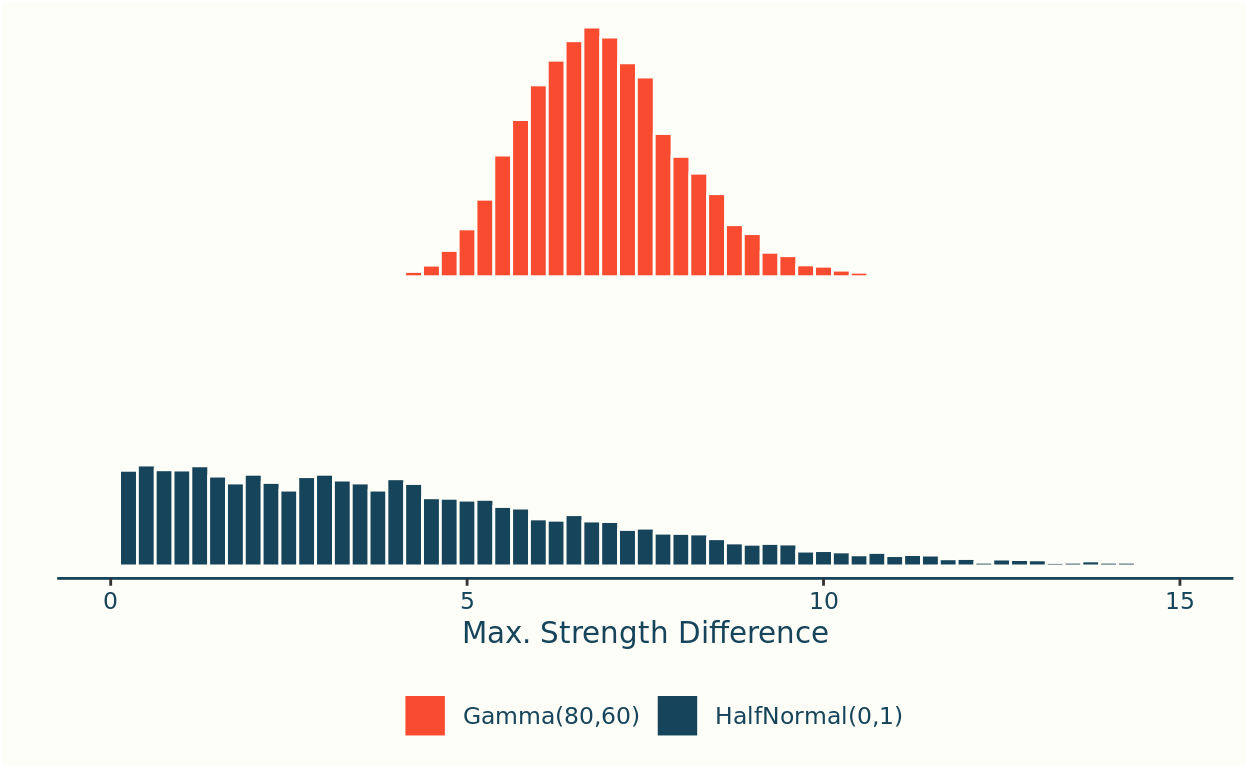This gives us the minor change to our original model specification:

BT2 \begin{align*} \bf{\text{Priors}}\\ \sigma & \sim \text{Gamma}(80,60) \\ \alpha_r & \sim \text{Normal}(0,\sigma) \\ \\ \bf{\text{Likelihood}}\\ \alpha_r - \alpha_s | W_{r,s} &\sim \text{logit}^{-1}(\alpha_r - \alpha_s) \end{align*}

Example Data
Show code
matches_sample <-  matches %>%
filter(event == '2020 UCI TRACK WORLD CYCLING CHAMPIONSHIPS', round == 'Finals', gender == 'Women') %>%
mutate(across(everything(), as.character)) %>%
select(event, date, gender, round, rider_1,rider_2,rider_id_1,rider_id_2, winner_id,loser_id) %>%
slice(1)

matches_sample <- tibble(Field = names(matches_sample), Example = unlist(matches_sample[1,]))
matches_sample %>%
kable("pipe") %>%
kable_styling(full_width=FALSE, bootstrap_options = c("striped", "hover", "condensed"),font_size = 10)

Field Example
event 2020 UCI TRACK WORLD CYCLING CHAMPIONSHIPS
date 2020-02-28
gender Women
round Finals
rider_1 HINZE EMMA
rider_2 VOINOVA ANASTASIIA
rider_id_1 44
rider_id_2 123
winner_id 44
loser_id 123

Stan code


functions {

real accuracy(vector delta, int start, int end){
vector[end - start + 1] delta_seg = segment(delta, start, end - start + 1);
real acc = 0;

for(n in 1:num_elements(delta_seg)) acc += (delta_seg[n] > 0);

return inv(num_elements(delta_seg)) * acc;
}

real log_loss(vector delta, int start, int end){
vector[end - start + 1] delta_seg = segment(delta, start, end - start + 1);
real ll = 0;

return inv(num_elements(delta_seg)) * bernoulli_logit_lpmf(1 | delta_seg);
}
}

data {
// Dimensions
int<lower=0> R; // Riders
int<lower=0> M; // Matches
int<lower=0,upper = M> T; // Training matches

// Match results
int<lower=1,upper=R> winner_id[M]; // ID's specifying riders in match
int<lower=1,upper=R> loser_id[M];
}

parameters {
// rider ratings
real<lower=0> sigma;
vector[R] alpha0;
}

transformed parameters {
// difference of winner and loser rating
vector[M] delta =alpha0[winner_id] - alpha0[loser_id];
}

model {
// (hierarchical) priors - strength
sigma ~ gamma(80,60);
alpha0 ~ normal(0,sigma);

// likelihood
}
generated quantities {
// maximum theoretical strength difference between two riders
real<lower=0> delta_max = max(alpha0) - min(alpha0);

// Calculate log-loss, match log-loss and accuracy. A separate value is returned
// for training/evaluation data, and within each of these the metrics are further
// broken down by round (5 rounds in total) and the total (6th entry in vectors).
real training_accuracy;
real evaluation_accuracy;
real training_log_loss;
real evaluation_log_loss;

training_accuracy = accuracy(delta,1, T);
training_log_loss = log_loss(delta, 1,T);
evaluation_accuracy = accuracy(delta, T+1, M);
evaluation_log_loss = log_loss(delta, T+1, M);
}

Visually the plot of posterior strengths looks pretty similar under the revised model to the original, so I’ll skip presenting it.

However, the change in prior does have a material impact on the maximum odds statistic, this is summarised in the table below which shows both the prior and posterior ranges for this statistic, for the two models.

Show code
bt2_summ <- read_remote_target("bt_summary_bt2_Women")

bt2_max_odds <- bt2_summ %>%
filter(str_detect(variable, 'delta_max')) %>%
select(q5, median, q95) %>%
mutate(
# calculation is based on the fact that the strength difference is the log odds
# on the exponential scale
across(everything(), ~format(round(10^(./log(10)),-2),big.mark = ","))
)

prior_max_odds <- max_diff %>%
mutate(model = if_else(hyperprior == 'Gamma(80,60)', 'bt2 - Prior', 'bt1 - Prior')) %>%
group_by(model) %>%
summarise(
q5 = quantile(10^odds_weaker_wins_log10, 0.05) %>% round(-1) %>% format(big.mark = ","),
median = quantile(10^odds_weaker_wins_log10,0.5) %>% round(-1) %>% format(big.mark = ","),
q95 = quantile(10^odds_weaker_wins_log10, 0.95) %>% round(-1) %>% format(big.mark = ",")
)

bind_rows(
bt1_max_odds %>% add_column(model = 'bt1 - Posterior', .before = 0),
bt2_max_odds %>% add_column(model = 'bt2 - Posterior', .before = 0),
prior_max_odds
)  %>%
mutate(
model = factor(model, levels = c("bt1 - Prior", "bt1 - Posterior", "bt2 - Prior", "bt2 - Posterior"))
)%>%
arrange(model) %>%
kable(col.names = c("", "5%", "50%", "95%"), digits = 2) %>%
kable_styling(full_width=FALSE)

5% 50% 95%
bt1 - Prior 0 40 32,950
bt1 - Posterior 1,500 12,000 211,900
bt2 - Prior 190 940 7,090
bt2 - Posterior 900 3,800 27,600

In both cases we can see that the posterior distributions are quite different from the priors. In the case of BT2 we can see that the extreme behaviour allowed by the naive prior choice has been reined in (even then its still much higher than my prior thought).

# Evaluation Metrics

With two models to hand, its time to consider how we can choose between the two; I’ll consider two formal evaluation metircs. Model accuracy answers the simple question If you always bet on the stronger athlete, how often would you win?. The log loss gives a more nuanced measure: penalising predictions when the model put a very low probability on the actual observed outcome.

\begin{align} \text{Accuracy} & = \text{Prop. of matches where the modelled stronger rider wins.} \\ \text{Log Loss} & = \text{Average log-probability assigned to the observed outcome.} \\ \end{align}

The definition of the log loss is equivalent to the log likelihood of the data, but divided by the number of data points so that we can more readily compare training/test performance.

As a benchmark for model performance we can compare to the model in which we randomly guess the winner. On average this algorithm would have an accuracy of $$\frac12$$ (as we’d expect half of its guesses to be correct), and the log loss is fixed at $$\log(\frac12) \approx -0.693$$.

Models with better predictive power will have higher accuracy (closer to 1), and higher log loss (closer to 0).

Accuracy
Show code
bt1_measures <- bt1_summ %>%
filter(str_detect(variable,'(accuracy|log_loss)')) %>%
extract(variable, into = c("split", "measure"), "(.*?)_(.*)") %>%
mutate(
model = 'bt1',
model_split = paste(model, "-", split)
)

bt2_measures <- bt2_summ %>%
filter(str_detect(variable,'(accuracy|log_loss)')) %>%
extract(variable, into = c("split", "measure"), "(.*?)_(.*)") %>%
mutate(
model = 'bt2',
model_split = paste(model, "-", split)
)

measures <- bind_rows(bt1_measures, bt2_measures)

Model Split 5% 50% (Median) 95%
bt1 evaluation 0.648 0.716 0.773
bt2 evaluation 0.648 0.716 0.773
bt1 training 0.770 0.791 0.810
bt2 training 0.764 0.787 0.807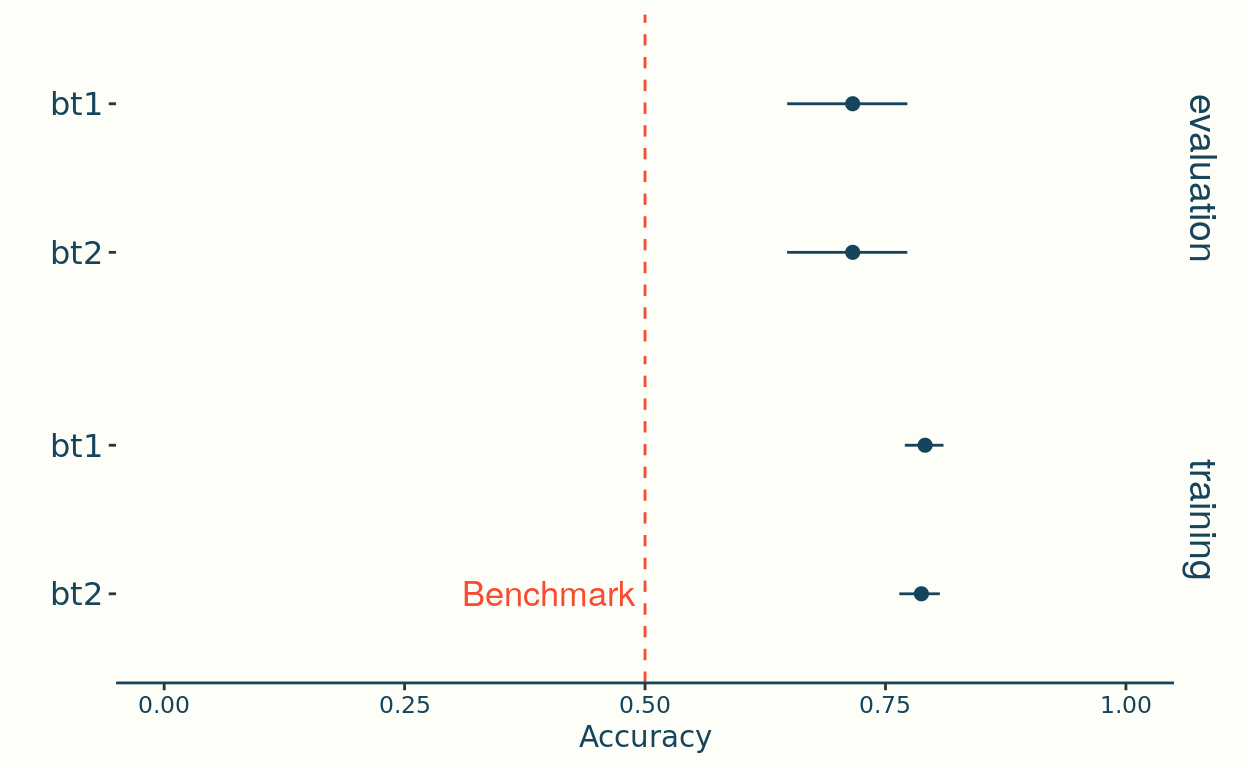Log Loss

Show code
log_loss <- measures %>%
filter(measure == "log_loss")

log_loss %>%
select(model, split,  q5, median, q95) %>%
arrange(split, model) %>%
kable("pipe", col.names =c("Model", "Split", "5%", "50% (Median)", "95%"), digits =3)

Model Split 5% 50% (Median) 95%
bt1 evaluation -0.780 -0.638 -0.536
bt2 evaluation -0.731 -0.619 -0.532
bt1 training -0.467 -0.440 -0.417
bt2 training -0.474 -0.450 -0.429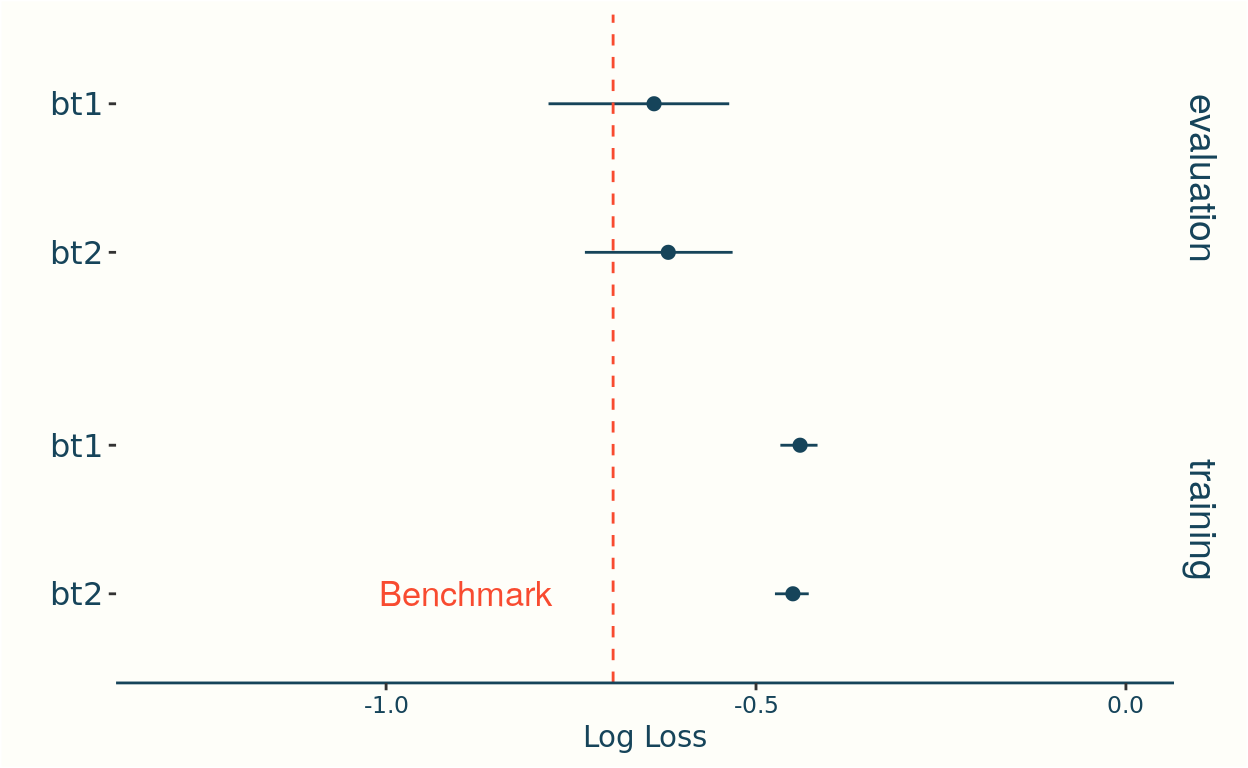Both models have accuracies that are well above the benchmark, indicating that using either model is better than random guessing, though there’s little distinction between the two models. This is to be expected as the accuracy will only change if the two models differ in their opinion about who the stronger rider is in each match, and we wouldn’t expect that to happen in many cases.

The log loss is more interesting: on the training data the log loss is better than guessing, but this isn’t the case in the evaluation data. Amongst the evaluation data, there’s some weak evidence that the Gamma prior (bt2) fairs marginally better.

This poor performance in log loss is driven by upsets: where the model is highly confident in one athlete winning, and then they don’t. For instance suppose a model thinks that a given athlete will win with probability 0.999, if they then fail to win that contributes a factor of $$\log(1 - 0.999) \approx -6.908$$ to the log loss, as opposed to if they win in which case the contribution is $$-0.001$$.

The Gamma prior fairs better as it was designed to lead the model away from scenarios where it offers astronomical odds.

# Next Steps

In this post I’ve introduced the basic Bradley-Terry model, and shown that it out performs a strategy of random guessing.

In the next post I’ll start to deviate from the standard model, accounting for features available in the data that should help to improve the predictive power of the model.